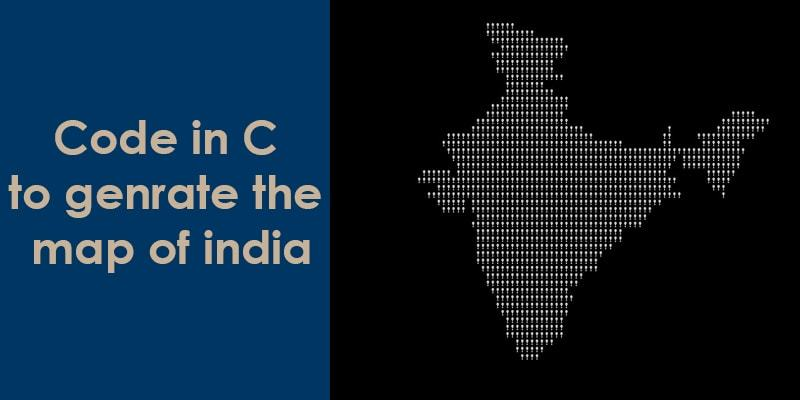## How to Create India Map Using C ProgramI just try googling and find a great source code to create India Map Using C Program. You think its not possible isn’t it !!

### Source Code Create India Map Using C Program:

```#include <stdio.h>
main()
{
int a,b,c;
int count = 1;
for (b=c=10;a="- FIGURE?, UMKC,XYZHello Folks,\
TFy!QJu ROo TNn(ROo)SLq SLq ULo+\
UHs UJq TNn*RPn/QPbEWS_JSWQAIJO^\
NBELPeHBFHT}TnALVlBLOFAkHFOuFETp\
HCStHAUFAgcEAelclcn^r^r\\tZvYxXy\
T|S~Pn SPm SOn TNn ULo0ULo#ULo-W\
Hq!WFs XDt!" [b+++21]; )
for(; a-- > 64 ; )
putchar ( ++c=='Z' ? c = c/ 9:33^b&1);
return 0;
}```

Updated :

If you’re Java developer and want to run this code in Java then check below code.

```public class IndiaMap
{
public static void main(String[] args) throws Exception
{
int a=10,b=0,c=10;
String s1="TFy!QJu ROo TNn(ROo)SLq SLq ULo+UHs UJq TNn*RPn/QPbEWS_JSWQAIJO^NBELPeHBFHT}TnALVlBLOFAkHFOuFETpHCStHAUFAgcEAelclcn^r^r\\tZvYxXyT|S~Pn SPm SOn TNn ULo0ULo#ULo-WHq!WFs XDt!";
a=s1.charAt(b);

while (a != 0)
{
if (b < 170)
{
a = s1.charAt(b);
b++;
while (a > 64)
{
a--;
if (++c=='Z')
{
c/=9;
System.out.print((char)(c));
}
else
System.out.print((char)(33 ^ (b & 0x01)));
}
}
else
break;
}
}
}```

### Output same for both program :

I am really curious that how it can be possible and try to find how this code work. And i got one answer on stackoverflow.

See other stuffHangprinter – Turns Any Room Into a 3D Printer

If you want to run this code online then like below for unlock the content:

The long string is simply a binary sequence converted to ASCII. The first `for` statement makes `b`start out at 10, and the `[b+++21]` after the string yields 31. Treating the string as an array, offset 31 is the start of the “real” data in the string (the second line in the code sample you provided). The rest of the code simply loops through the bit sequence, converting the 1’s and 0’s to !’s and whitespace and printing one character at a time.

I think you should see thisWorld’s worst song, the 62-year-old Ban

#### Less obfuscated version:

```#include "stdio.h"
int main (void) {
int a=10, b=0, c=10;
char* bits ="TFy!QJu ROo TNn(ROo)SLq SLq ULo+UHs UJq TNn*RPn/QPbEWS_JSWQAIJO^NBELPeHBFHT}TnALVlBLOFAkHFOuFETpHCStHAUFAgcEAelclcn^r^r\\tZvYxXyT|S~Pn SPm SOn TNn ULo0ULo#ULo-WHq!WFs XDt!";
a = bits[b];
while (a != 0) {
a = bits[b];
b++;
while (a > 64) {
a--;
if (++c == 'Z') {
c /= 9;
putchar(c);
} else {
putchar(33 ^ (b & 0x01));
}
}
}
return 0;
}```

The clever part is in the `putchar` statements. Take the first `putchar`. ASCII `'Z'` is 90 in decimal, so 90 / 9 = 10 which is a newline character. In the second, decimal 33 is ASCII for `'!'`. Toggling the low-order bit of 33 gives you 32, which is ASCII for a space. This causes `!` to be printed if `b` is odd, and a blank space to be printed if `b` is even. The rest of the code is simply there to walk the “pointer” `a` through the string.

If you’ve also an answer of this then comment below.#### By Tell Me How

It is a technology blog and admin has excellent experience in programming from 5+ year. You can contact us at ceo.tellmehow@gmail.com

#### How to Clean Vegetable and Fruits from Coronavirus Infection?